# Write the trigonometric expression in terms of sine and cosine, and then simplify.cos(u) + sin(u) tan(u)

Question
1 views

Need help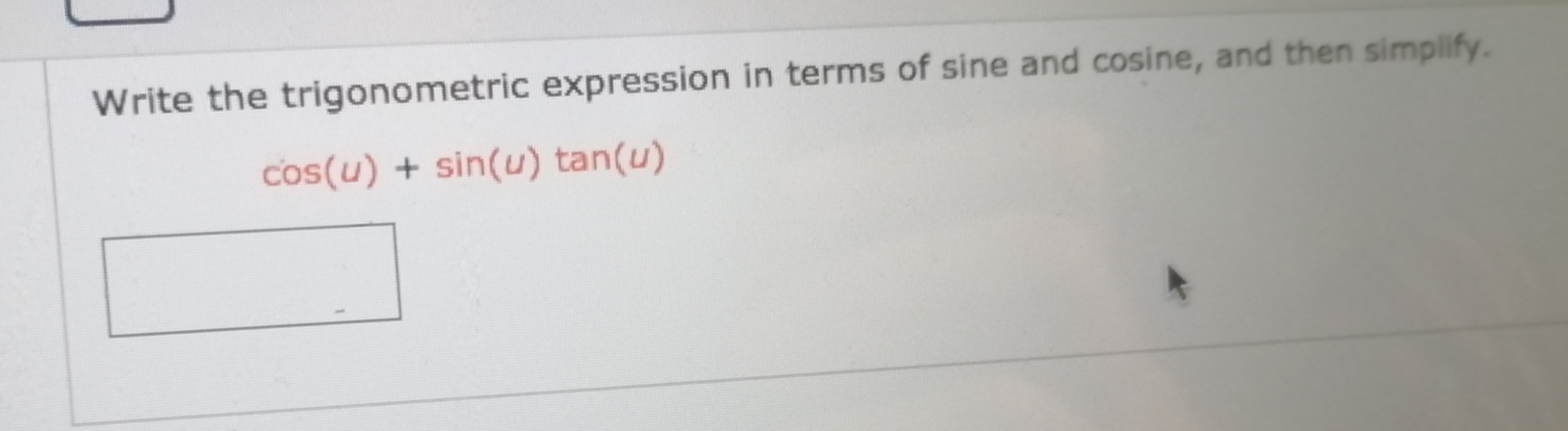help_outlineImage TranscriptioncloseWrite the trigonometric expression in terms of sine and cosine, and then simplify. cos(u) + sin(u) tan(u) fullscreen
check_circle

Step 1

Given trigonometric expression is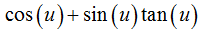Step 2

Use the below trigonometric identity to rewrite the given expression in terms of since and cosine.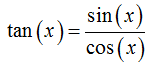Plug it in above expression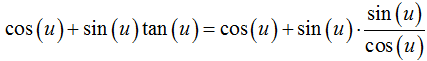Step 3

Simplify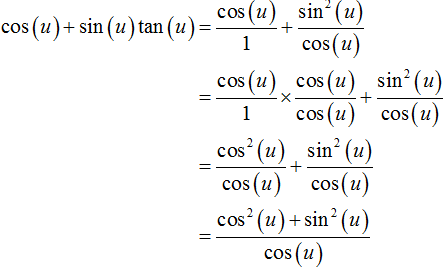...

### Want to see the full answer?

See Solution

#### Want to see this answer and more?

Solutions are written by subject experts who are available 24/7. Questions are typically answered within 1 hour.*

See Solution
*Response times may vary by subject and question.
Tagged in